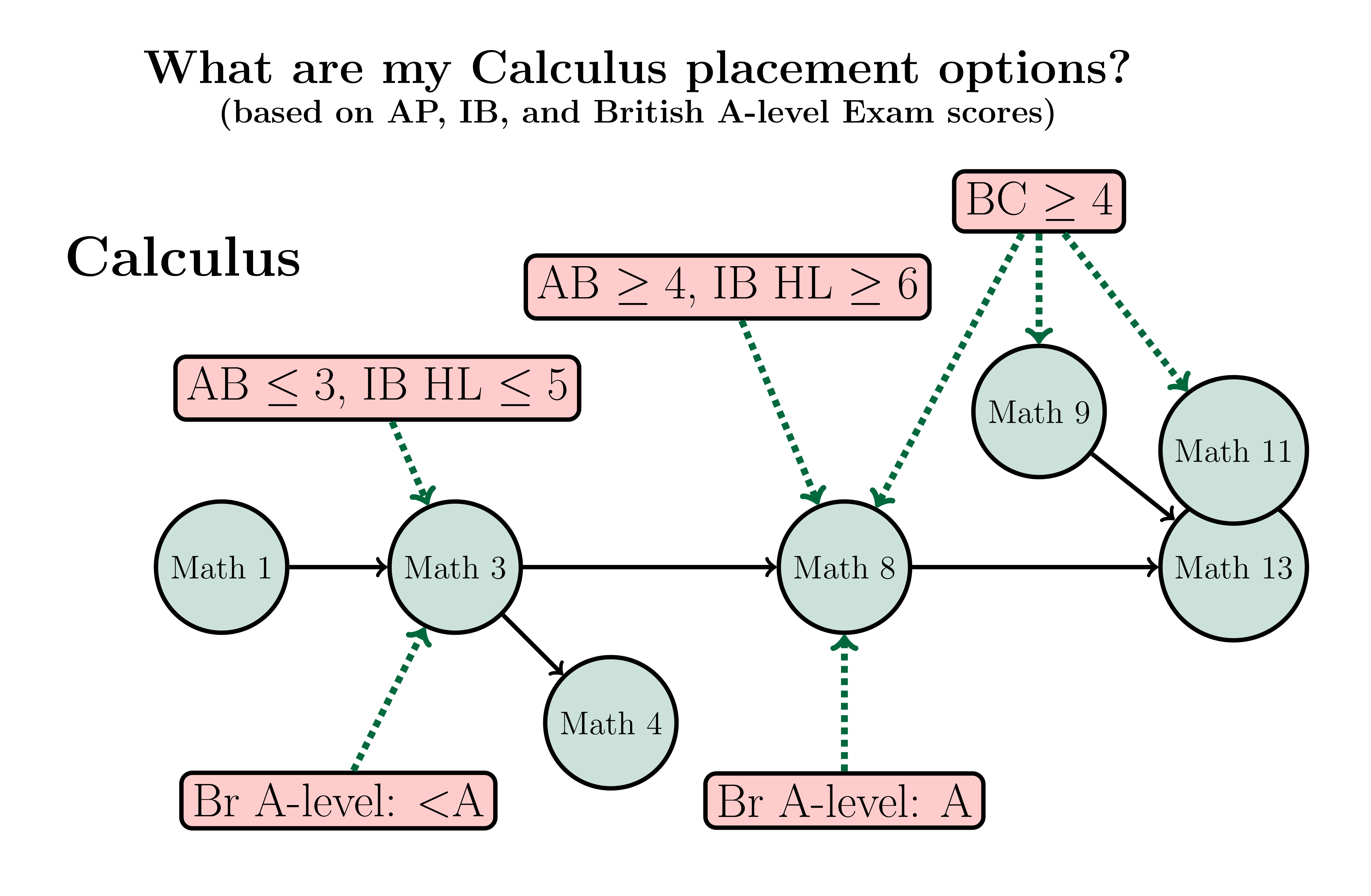This website uses features that are not well-supported by your browser. Please consider upgrading to a browser and version that fully supports CSS Grid and the CSS Flexible Box Layout Module.
Dartmouth Mathematics Placement System## Core Calculus Courses and Variants

• To accommodate the diverse backgrounds of our entering students, single and multivariable calculus courses have multiple entry points. In all, there are four (core) calculus courses (Math 1, 3, 8, 13) spanning single and multivariable calculus, and two more hybrid courses (9 and 11) which offer variations to the core sequence.
• We shall describe in words and in more detail the content of the aforementioned courses and for whom they are appropriate, but the following diagram will perhaps allow you to focus more quickly on what exactly you want to read:#### The Core Calculus Courses

The standard calculus sequence, Math $1\to3\to 8\to 13$, is the main spine of the diagram above. Where and how you join the core sequence is the subject of this web page.

#### Variations on the core sequence

Students who have placement credit for both Math 3 and Math 8 via either the Advanced Placement BC exam, or by having passed the department's Math 8 credit exam, have several options to consider if they wish to continue along the calculus track to multivariable calculus.

The Issue: A typical AB/BC syllabus is covered (at Dartmouth) by taking Math 3 and the first half of Math 8. The second half of Math 8 investigates differential multivariable calculus — loosely speaking, the general themes of AB calculus, but for functions of several variables.

The Options: The option you choose depends solely on your interests and your level of confidence in your command of the material covered in previous calculus courses. Let's repeat that point: The choice is entirely yours.

Option 1 (Math 11): If you are very confident in your knowledge of material on the BC syllabus, and are up for a briskly paced course, then Math 11 (Calculus for students with two terms of advanced placement credit) would be a good choice for you (a typical Math 11 syllabus) . This course covers the material from the second half of Math 8 on the differential aspects of multivariable calculus, while also covering the integration theory of Math 13. At the end of this course, you will have the equivalent of Math 13, and can take any course for which Math 13 is listed as a prerequisite. Your next step is most likely a 20's level course, perhaps linear algebra or differential equations.

Option 2 (Math 9): A second option is for those who are confident in their knowledge of the BC syllabus material so do not want to repeat it, yet want to move forward at a less brisk pace than offered by Math 11. The course Math 9 (Multivariable Differential Calculus with Linear Algebra) offers the material from the second half of Math 8, but enhanced with a brief introduction to linear algebra so as to better explain how derivatives of functions of several variables are linear approximations to functions (a sample Math 9 syllabus). Following Math 9, the next course in the calculus sequence would be Math 13.

Option 3 (Math 13): If you have obtained credit for Math 3 and 8, and have taken some multivariable calculus in high school (in particular the topics covered in Dartmouth's Math 8 (check recent Math 8 syllabi )), you will likely be fine taking Math 13 instead of Math 11. You should confirm this with the adviser to first-year students.

Option 4 (Math 8): On the other hand, if you are tentative about your skills on the later parts of the BC syllabus, e.g., techniques of integration and infinite series, you could (even though you officially have credit for it), opt to retake Math 8 (Calculus of Functions of One and Several Variables). This is a seldom chosen option, but if you do choose this, the Math 8 AP credit on your transcript will be replaced by your eventual grade in the Math 8 course you take here. This option offers a chance to strengthen areas from the BC syllabus in the first half of the course, while then advancing to introduce the differential multivariable calculus (check recent Math 8 syllabi ). Following Math 8, the next course in the calculus sequence would be Math 13.